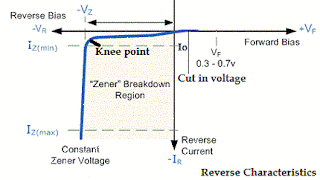## Search This Blog

### Zener diode characteristics

The VI characteristics of Zener diodes shown in the figure given below. This curve shows that when Zener diode if forward bias, it works like a normal diode but when the reverse biased voltage applies across to the Zener diode, the Zener diode breakdown occurs in a different manner.

At Zener breakdown voltage the current stary flowing in the reverse direction, it allows only a small amount of leakage current until the voltage is less than Zener diode
At the certain value of reverse voltage, the reverse current will increase suddenly and sharply. This is an indication that the breakdown has occurred. Breakdown occurs called a Zener breakdown. However, the Zener diode exhibits a controlled breakdown that does damage the device.

This graph shows that Zener breakdown is not exactly vertical shown above which shows that the Zener diode has a resistance. This breakdown voltage of the Zener diode depends on the amount of doped applied. If the diode has heavily doped Zener breakdown occurs at a low reverse voltage, on a different way when the diode is lightly doped breakdown occurs at high reverse voltage. The voltage across Zener is represented by the equation is given below.

V = Vz+IzRzZener diode characteristics
The VI characteristics of Zener diodes shown in the figure given below. This curve shows that when Zener diode if forward bias, it works like a normal diode but when the reverse biased voltage applies across to the Zener diode, the Zener diode breakdown occurs in a different manner.

At Zener breakdown voltage the current stary flowing in the reverse direction, it allows only a small amount of leakage current until the voltage is less than Zener diode
At the certain value of reverse voltage, the reverse current will increase suddenly and sharply. This is an indication that the breakdown has occurred. Breakdown occurs called a Zener breakdown. However, the Zener diode exhibits a controlled breakdown that does damage the device.

This graph shows that Zener breakdown is not exactly vertical shown above which shows that the Zener diode has a resistance. This breakdown voltage of the Zener diode depends on the amount of doped applied. If the diode has heavily doped Zener breakdown occurs at a low reverse voltage, on a different way when the diode is lightly doped breakdown occurs at high reverse voltage. The voltage across Zener is represented by the equation is given below.

V = Vz+IzRzZener diode characteristics